# Boys and glasses

2/3 of the students in Sarah’s class are boys. Of the boys, 1/3  of them wear glasses. What fraction of the students wear glasses?

x =  0.2222

### Step-by-step explanation:Did you find an error or inaccuracy? Feel free to write us. Thank you!Tips to related online calculators
Need help to calculate sum, simplify or multiply fractions? Try our fraction calculator.
Check out our ratio calculator.

## Related math problems and questions:

• Glasses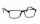There are 36 pupils in the class. Nine girls wear glasses. Boys with glasses are five less than girls without glasses. Boys without glasses are two times more than girls without glasses. How many boys and how many girls?
• The ratioThe ratio of girls to boys in Mrs. White's class is 3:2.  If there are 12 boys, how many girls are in the classroom?
• Boys and girls 8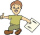In a class of 35 boys and girls that has a ratio of 2:5, 5 boys are absent and 5 boys are present. How many students are present?
• SarahSarah has a photography blog. 3/7 of her photos are of nature, 1/4 of the rest are of her friends. What fraction of all of Sarah's photos is of her friends?
• A class IV.AIn a class there are 15 girls and 30 boys.  What fraction of the class represents the boys?
• The classroom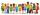In the seventh class the number of boys and girls are at a ratio of 4:5. Boys are 16. How many total students are in the classroom?
• Prospering studentsThere are girls and 30 boys in the class. Boys prospering 28, girls all. How many girls are in the class and how many boys are there, if all the prospering students are 95%?
• FortyForty five of the 80 students were girls. What is the ratio of girls to boys?
• One third 2One third of all students in class live in a house. If here are 42 students in a class, how many of them live in house?
• PupilsThere are 32 pupils in the classroom, and girls are two-thirds more than boys. a) How many percents are more girls than boys? Round the result to a whole percentage. b) How many boys are in the class? c) Find the ratio of boys and girls in the class. Writ
• Scouts 4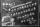4/7 of the students in a school are boys. If 3/8 of the boys are scouts, how many scouts are there in a school of 1878 students?
• English and math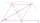1/5 of a class study English, and 3/5 study mathematics. If the class consist of 80 students, how many students study English and mathematics in the class?
• Pupils 7There are 40 pupils in a certain class. 3/5 of the class are boys. How many are girls?
• There 12There are 42 students in the class and 2/3 of them are girls. How may girls are there in the class?
• Boys and girlsIf the ratio of male & female teachers is 2/5 & it is in proportion of the male & female students, how many are girls if there are 42 boys?
• School trip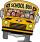School trip cost 247.2 Eur for one class (24 students). How much would a trip cost for two classes? (both classes together have 53 students)
• Students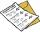The class has 22 students. 2 of which have assessments of 2. The count of assessment of 1 is three times more than the count of students with an assessment of 3. How many students have an assessment of 1, and how many students have an assessment of 1.